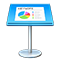# 鍵盤快速鍵

Control + B

Control + F

Option + 向左鍵或 Option + Control + B

Option + 向右鍵或 Option + Control + F

Command + 向上鍵

Command + 向下鍵

Command + 向左鍵

Command + 向右鍵

Option + 向上鍵

Option + 向下鍵

Command + E

Command + J

Home

End

Control + L

Page Down

Page Up

Home

End

Command + A

Shift + Command + A

Shift + 向右鍵

Shift + 向左鍵

Option + Shift + 向右鍵

Option + Shift + 向左鍵

Shift + Command + 向右鍵

Shift + Command + 向左鍵

Shift + 向上鍵

Shift + 向下鍵

Option + Shift + 向上鍵

Option + Shift + 向下鍵

Shift + Command + 向上鍵或 Shift + Home

Shift + Command + 向下鍵或 Shift + End

Command + T

Shift + Command + C

Command + B

Command + I

Command + U

Delete

Option + Delete

Option + 向前刪除（並非所有鍵盤皆適用）

Control + K

Command + 加號（+）

Command + 減號（–）

Option + Command + 左中括號（[）

Option + Command + 右中括號（]）

Control + Shift + Command + 加號（+）

Control + Command + 減號（–）

Command + 左大括號（{）

Command + 垂直線（|）

Command + 右大括號（}）

Option + Command + 垂直線（|）

Command + 左中括號（[）

Command + 右中括號（]）

Shift + Tab

Tab

Command + K

Command + X

Command + C

Option + Command + C

Command + V

Option + Command + V

Option + Shift + Command + V

Option + 空白鍵

Shift + Return

Return

Control + O

Control + Command + 空白鍵

Control + T

Command + F

Command + G

Shift + Command + G

Command + E

Return

Command + J

Esc

Control + Command + D

Option + Esc

Command + 分號（;）

Shift + Command + 分號（:）

Shift + Command + K

Option + Command + K

Option + Shift + Command + K

Tab

Shift + Tab

Option + Command + C

Option + Command + V

Shift + Command + B

Option + Shift + Command + B

Shift + Command + F

Option + Shift + Command + F

Option + Command + G

Option + Shift + Command + G

Command + L

Option + Command + L

Command + D 或按住 Option 並拖移

Shift + Command + M

Return、Enter 或按兩下

Command + Return

Control + Option + Command + I

Control + Option + Command + T

Option + 向上鍵

Option + 向下鍵

Option + 向右鍵

Option + 向左鍵

Shift + 向上鍵或 Shift + 向下鍵

Shift + 向右鍵或 Shift + 向左鍵

Command + A

Delete

Option + Command + C

Option + Command + V

Control + Shift + Command + V

Option + Command + U

Command + Return

Tab

Shift + Tab

Return

Shift + Return

Command + Return

Option + Tab

Control + Return

Return

Shift + Command + V

Return 或 Tab

Esc（Escape）

Shift + Command + D

Return

Shift + Return

Tab

Option + Return

Option + Tab

Command + N

Enter

Command + O

Esc

Command + S

Option + Shift + Command + S

Shift + Command + S

Command + P

Command + Shift + 問號（?）

Command + W

Option + Command + W

Command + M

Option + Command + M

Command + Control + F

Command + 右角括號（>）

Command + 左角括號（<）

Command + 逗號（,）

Shift + Command + 0

Command + 0

Command + R

Shift + Command + V

Command + Shift + C

Command + Option + T

Option + Command + I

Control + Grave（`）

Shift + Control + Grave（`）

Command + H

Command + Option + H

Command + Z

Command + Shift + Z

Command + Q

Option + Command + Q

Option + Shift + Command + P

Return 或 Shift + Command + N

Tab

Shift + Tab

Command + D

Delete

Shift + Command + H

Shift + 向右鍵

Shift + 向左鍵

Shift + Command + 向上鍵

Shift + Command + 向下鍵

Command + 向上鍵

Command + 向下鍵

Option + Command + P

Shift + 向右鍵

Shift + 向下鍵或 Shift + Page Down

Shift + 向左鍵或 Shift + Page Up

Z

F

B

W

C

S

Return

Esc

X

R

U

D

Command + 加號

Command + 減號

Esc 或 Q

H

home

end

K

J

L

L

O